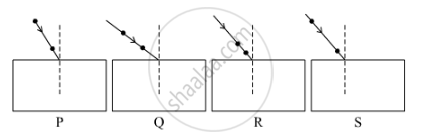# Select from the Following the Best Experimental Setup for Tracing the Path of a Ray of Light Passing Through a Rectangular Glass Slab. - Science

Select from the following the best experimental setup for tracing the path of a ray of light passing through a rectangular glass slab.(a) P

(b) Q

(c) R

(d) S

#### Solution

Among the given options, S will be the most suitable setup for tracing a ray of light passing through a rectangular glass slab.

Hence, the correct answer is option (d).

Concept: Refraction of Light Through a Rectangular Glass Slab
Is there an error in this question or solution?